# Modern Physics Practice Level - 1

## 45 Questions MCQ Test Exclusive Video Lectures of Class 12 Physics by Experts | Modern Physics Practice Level - 1

Description
This mock test of Modern Physics Practice Level - 1 for Class 12 helps you for every Class 12 entrance exam. This contains 45 Multiple Choice Questions for Class 12 Modern Physics Practice Level - 1 (mcq) to study with solutions a complete question bank. The solved questions answers in this Modern Physics Practice Level - 1 quiz give you a good mix of easy questions and tough questions. Class 12 students definitely take this Modern Physics Practice Level - 1 exercise for a better result in the exam. You can find other Modern Physics Practice Level - 1 extra questions, long questions & short questions for Class 12 on EduRev as well by searching above.
QUESTION: 1

Solution:
QUESTION: 2

Solution:
QUESTION: 3

### Light of wavelength 0.6 μm from a sodium lamp fallson a photocell and causes the emission of photoelectrons for which the stopping potential is 0.5V. With light of wavelength 0.04 μm from a mercury vapor lamp, the stopping potential is 1.5 V. Then, the work function [In electron volts] of the photocell surface is

Solution:
QUESTION: 4

Ultraviolet light of wavelength 300 nm and intensity 1.0 Wm-2 falls on the surface of a photosensitive material. If one per cent of the incident photons produce photo-electrons, then the number of photoelectrons emitted per second from an area of 1.0 cm2 of the surface is nearly

Solution:
QUESTION: 5

In a photocell, with excitation wavelengthl , the fastselectron has speed v. If the excitation wavelength ischanged to 3λ /4, the speed of the fastest electron will be

Solution:
QUESTION: 6

Two identical photocathodes receive light of frequenciesf1and f2. If the velocities of the photoelectrons (of massmi coming out are v1 and v2, respectively, then

Solution:
QUESTION: 7

The work function of a metal is W and λ is the wave length of the incident radiation. There is no emission of photoelectrons when

Solution:
QUESTION: 8

Monochromatic light incident on a metal surface emitselectrons with kinetic energies from zero to 2.6 eV.What is the least energy of the incident photon if thetightly bound electron needs 4.2 eV to remove?

Solution:
QUESTION: 9

The work function for sodium surface is 2.0 eV andthat for aluminium surface is 4.2 eV. The two metalsare illuminated with appropriate radiations so as tocause photoemission. Then

Solution:
QUESTION: 10

Representing the stopping potential V along y-axis and(1/ λ ) along x-axis for a given photocathode, the curveis a straight line, the slope of which is equal to

Solution:
QUESTION: 11

Electrons traveling at a velocity of 2.4 x 106 ms-1 entera region of crossed electric and magnetic fields shownin Fig. If the electric field is 3.0 x 106 Vm and the fluxdensity of the magnetic field is 1.5 T, the electrons upon entering the region of the crossed fields will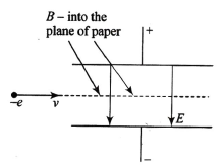Solution:
QUESTION: 12

A surface irradiated with light λ = 480 nm gives outelectrons with maximum velocity v m/s. the cin offwavelength being 600 nm. The same surface wouldrelease electrons with maximum velocity 2v m/s if it isirradiated by light of wavelength.

Solution:
QUESTION: 13

ln an experiment on the photoelectric effect, an evacuated photocell with a pure metal cathode is used. Which graph best represents the variation of V, the minimum potential difference needed to prevent current from flowing, when x. the frequency of the incident light, is varied ?

Solution:
QUESTION: 14

A metal surface in an evacuated tube is illuminated with monochromatic light causing the emission of photoelectrcss which are collected at an adjacent electrode. For a giwen intensity of light, the way in which the photocurrent I depends in the potential difference Vbetween the electrodes is shown by approximate graph in Fig. If the experiment were repeated with light of twice the intensity but the same wavelength, which of the graphs below would best represent the new relation between I and VI (In these graphs, the result of the original experiment is indicated by a broken line.)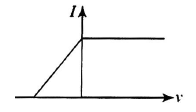Solution:
QUESTION: 15

If stopping potentials corresponding to wavelengths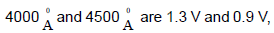respectively, then the work function of the

Solution:
QUESTION: 16

The frequency and the intensity of a beam of light fallingon he surface of a photoelectric material are increasedby a factor of two. This will

Solution:
QUESTION: 17

The frequency of incident light falling on a photosensitivemetal plate is doubled, the KE of the emitted photoelectrons is

Solution:
QUESTION: 18

Lights of two different frequencies whose photons havey energies 1 and 2.5 eV, respectively, successivelyilluminate a metal whose work function is 0.5 eV. Theratio of the maximum speeds of the emitted electronswill be

Solution:
QUESTION: 19

A cesium photocell, with a steady potential differenceof 60 V across it, is illuminated by a small bright lightplaced 1 m away. When the same light is placed 2 maway, the electrons crossing the photocell

Solution:
QUESTION: 20

If 5% of the energy supplied to a bulb is irradiated asvisible light, how many quanta are emitted per secondby a 100 W lamp? Assume wavelength of visible lightas 5.6 x 10-5 cm

Solution:
QUESTION: 21

Which of the following graphs correctly represents the variation of particle momentum with associated de Broglis wavelength ?

Solution:
QUESTION: 22

A point source causes photoelectric effect from a smallmetal plate. Which of the curves in Fig.may representthe saturation photo-current as a function of the distancebetween the source and the metal?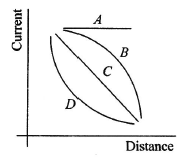Solution:
QUESTION: 23

Let p and E denote the linear momentum and energy,respectively, of a photon. If the wavelength is decreased,

Solution:
QUESTION: 24

The human eye can barely detect a yellow light (λ = 6000 Å) delivers 1.7 x 10-18 W to the retina. The number of photons per second falling on the eye is nearest to

Solution:
QUESTION: 25

An electron is accelerated through a potential differenceof 200 V. If e/m for the electron be 1.6 x 1011coulomb kg-1, then the velocity acquired by the electron will be

Solution:
QUESTION: 26

A photoelectric cell is connected to a source of variablepotential difference, connected across it and thephotoelectric current resulting (μA) is plotted againstthe applied potential difference (V). The graph in thebroken line represents one for a given frequency andintensity of the incident radiation. If the frequency isincreased and the intensity is reduced, which of thefollowing graphs of unbroken line represents the newsituation?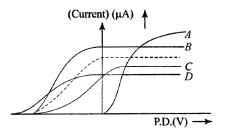Solution:
QUESTION: 27

An electron of mass me and a proton of mass mp areaccelerated through the same potential difference. Theratio of the de Broglie wavelength associated with anelectron to that associated with proton is

Solution:
QUESTION: 28

A material particle with a rest mass mo is moving withvelocity of light c. Then, the wavelength of the de Brogliewave associated with it is

Solution:
QUESTION: 29

Out of a photon and an electron, the equation E = pc, is valid for

Solution:
QUESTION: 30

The ratio of momenta of an electron and an a -particlewhich are accelerated from rest by a potential differenceof 100 V is :

Solution:
QUESTION: 31

The kinetic energy of an electron is E when the incident wavelength is λ . To increase the KE of the electron to 2E. the incident wavelength must be

Solution:
QUESTION: 32

The potential difference applied to an X-ray tube is V.The ratio of the de Broglie wavelength of electron to theminimum wavelength of X-ray is directly proportional to

Solution:
QUESTION: 33

A monochromatic source of light is placed at a largedistance d from a metal surface. Photoelectrons areejected ix at rate n, the kinetic energy being E. If thesource is brought nearer to distance d/2, the rate and kinetic energy per photoelectron become nearly

Solution:
QUESTION: 34

A nozzle throws a stream of gas against a wall with avelocity v much larger than the thermal agitation of themolecules. The wall deflects the molecules withoutchanging the magnitude of their velocity. Also, assumethat the force exerted on the wall by the molecules isperpendicular to the wall. (This is not strictly true for arough wall). Find the force exerted on the wall.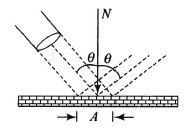Solution:
QUESTION: 35

What is the de Broglie wavelength of the waveassociated with an electron that has been acceleratedthrough s potential difference of 50.0 V?

Solution:
QUESTION: 36

In a photoelectric effect, electrons are emitted

Solution:
QUESTION: 37

A particle of mass 'm' is projected from ground with velocity u making an angle 'θ' with the vertical. The de-Broglie wavelength of the particle at the highest point is :

Solution:
QUESTION: 38

Two identical non-relativistic particles A and B move atright angles to each other, processing de Brogliewavelengths λ1 and λ2  respectively. The de Brogliewavelength of each particle in their centre of mass frameof reference is

Solution:
QUESTION: 39

With respect to Electromagnetic Theory of Light, thePhotoelectric effect is best explained by statement

Solution:
QUESTION: 40

Two electrons are moving with same speed v. Oneeternal enters a region of uniform electric field whilethe othe enters a region of uniform magnetic field, thenafter soot time de Broglie wavelengths of two are λ1and λ2 :. respectively. Now,

Solution:
QUESTION: 41

A sensor is exposed for time t to a lamp of power P placed at a distance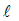. The sensor has an openingthat is 4d a diameter. Assuming all energy of the lampis given off a light, the number of photons entering thesensor if wavelength of light is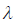is

Solution:
QUESTION: 42

Let E1 and E2 be the binding energies of two nuclei A and B. is observed that two nuclei of A combine together toform a B nucleus. This observation is correct only if

Solution:
QUESTION: 43

A radioactive sample decays by 63% of its initial value in10 s. It would have decayed by 50% of its initial value in

Solution:
QUESTION: 44

A nucleus moving with velocity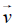emits an α - particle. Let the velocities of the α -particle and the remaining nucleus be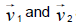and their masses be m1 and m2, then

Solution:
QUESTION: 45

Which of the following statements is incorrect for nuclear forces?

Solution: